# Cardiac arrhythmia classification using autoregressive modeling

## Abstract

### Background

Computer-assisted arrhythmia recognition is critical for the management of cardiac disorders. Various techniques have been utilized to classify arrhythmias. Generally, these techniques classify two or three arrhythmias or have significantly large processing times. A simpler autoregressive modeling (AR) technique is proposed to classify normal sinus rhythm (NSR) and various cardiac arrhythmias including atrial premature contraction (APC), premature ventricular contraction (PVC), superventricular tachycardia (SVT), ventricular tachycardia (VT) and ventricular fibrillation (VF).

### Methods

AR Modeling was performed on ECG data from normal sinus rhythm as well as various arrhythmias. The AR coefficients were computed using Burg's algorithm. The AR coefficients were classified using a generalized linear model (GLM) based algorithm in various stages.

### Results

AR modeling results showed that an order of four was sufficient for modeling the ECG signals. The accuracy of detecting NSR, APC, PVC, SVT, VT and VF were 93.2% to 100% using the GLM based classification algorithm.

### Conclusion

The results show that AR modeling is useful for the classification of cardiac arrhythmias, with reasonably high accuracies. Further validation of the proposed technique will yield acceptable results for clinical implementation.

## Background

Automatic ECG analysis is critical for diagnosis and treatment of critically ill patients. Modeling and simulation of ECG under various conditions are very important in understanding the functioning of the cardiovascular system as well as in the diagnosis of heart diseases. Arrhythmias represent a serious threat to the patient recovering from acute myocardial infarction, especially ventricular arrhythmias like ventricular tachycardia (VT) and ventricular fibrillation (VF). In particular, VT and VF are life-threatening conditions and produce significant haemodynamic deterioration . There is a need for quick identification of these conditions. Other arrhythmias like atrial premature contraction (APC), premature ventricular contraction (PVC) and superventricular tachycardia (SVT) are not as lethal as VF, but are important in diagnosing the disorders of the heart. The reliable detection of these arrhythmias constitutes a challenge for a cardiovascular diagnostic system. Consequently, significant amount of research has focused on the development of algorithms for accurate diagnosis of ventricular arrhythmias.

Various studies have been performed to classify various cardiac arrhythmias . A number of techniques have been used for identification of arrhythmias including correction waveform analysis , time-frequency analysis , complexity measures , and a total least squares-based Prony modeling algorithm . Different features are extracted from the ECG for classification of ventricular arrhythmias including QRS and ST segment based values, heart rate, spectral features, AR coefficients, complexity measures and nonlinear measures .

Cardiac arrhythmias using two intracardiac channels can be detected using the correlation waveform analysis (CWA) . CWA was used to detect morphologic changes in the intracardiac electrogram when compared to electrograms during sinus rhythm. Each electrogram had its respective template and the templates were obtained by signal averaging the waveform from a passage of sinus rhythm. The software trigger was used to align the template with the cycle being tested. A window size of N point defined each cycle.

Methods such as direct ECG feature detection , Fourier transform , time-frequency analysis  and complexity analysis  have also been employed. The main objective of the direct ECG feature detection was to investigate how many different ventricular conduction defects (VCD) categories can be formed by advanced cluster analysis methods, which reduce the number of classification parameters into a reasonably small set for a meaningful classification. The second objective was to investigate to what extent a select set of repolarization parameters would help in identification of distinct VCD subgroups. The study of detection of ECG features show that the key features of ECG are QRS duration, T axis angle, T amplitude, QRS axis angle and spatial angle. Typically, morphological features related to the P, QRS and ST waves are used for ECG signal analysis .

A technique based on averaged threshold crossing intervals was proposed for the detection of VT and VF based on heart rate measurements . A modified sequential detection algorithm was further proposed to improve the accuracy of detecting VT and VF . A Fourier transform based algorithm has been proposed for the detection of supraventricular rhythms from ventricular rhythms . Power spectra computed from the QRS complexes extracted from ECG signals were classified using a neural network. High sensitivity and specificity values greater than 98% have been reported for discriminating supraventricular rhythms from ventricular rhythms. However, SVT and VT were grouped together as ventricular rhythms. A new algorithm based on complexity measures was proposed for the detection of NSR, VT and VF . The algorithm was tested for varying lengths of data and very high accuracy values were achieved for data lengths of 7 sec for classifying NSR, VT and VF. The algorithm was suggested for real-time implementation in automatic external defibrillators.

Recently, a new approach for the discrimination among VF, VT and SVT has been developed using a total least squares-based Prony modeling algorithm . Two features, energy fractional factor (EFF) and predominant frequency (PF) were derived from the total least squares based Prony model. A two-stage classification method is used in which the EFF is used for discriminating SVT from VT and VF in the first stage followed by using PF for further separation of VF and VT the second stage. A classification accuracy of 95.24%, 96.00% and 97.78% were reported for SVT, VF and VT respectively for the Prony modeling algorithm. However, the total least squares-based Prony modeling technique did not consider NSR, APC and PVC for feature extraction and discrimination.

AR modeling has been used extensively to model heart rate variability (HRV) and for power spectrum estimation of ECG and HRV signals . Amplitude modulated sinusoidal signal model, which is a special case of the time-dependent AR model have been applied to modeling ECG signals . Adaptive AR modeling with Kalman filtering has been used . Parameters extracted from AR modeling have been used for arrhythmia classification in conjunction with other features . For example, two AR coefficients, along with the mean-square value of the QRS complex segments were utilized as features for classification of normal and abnormal PVC, where the prediction order was only 2, a fuzzy adaptive resonance theory mapping (ARTMAP) was used for classification. The best result of PVC correct detection were 92% under the ratios of the training data size and testing data size was 2 to 4 . It has been suggested that increasing the model order would not reduce the prediction error implying that a linear predictor order of two is sufficient for fast cardiac arrhythmia detection .

The objective of the present study is to model the ECG signal and classify certain cardiac arrhythmias at the ICU. Most of the techniques involve significant amounts of computation and processing time for extraction of features and classification. The other disadvantage is the small number of arrhythmias classified using a given technique with most techniques being used to classify two to three arrhythmias [2, 413, 15, 16]. There is a need for extending a particular technique for a larger number of arrhythmias. In addition, the proposed technique should be amenable to real-time implementation so that it can be used in intensive care units.

In this study, the ECG signals were modeled using AR analysis for classifying cardiac arrhythmias. The advantage of AR modeling is its simplicity and it is suitable for real-time classification at the ICU or ambulatory monitoring. AR models are popular due to the linear form of the system of simultaneous equations involving the unknown AR model parameters and the availability of efficient algorithm for computing the solution [23, 24]. AR modeling has been used in various applications including classification of physiological signals like electroencephalograms . AR modeling is adapted for extracting good features from ECG signals, thus enabling the discrimination of certain ECG arrhythmias. The computed AR coefficients were checked for modeling accuracy for the various types of ECG signals. Various pattern classification techniques have been applied to the classification of arrhythmias [3, 12, 14, 16, 2628]. In the current study, the AR coefficients computed from the ECG signals were classified using a generalized linear model (GLM) . Various arrhythmias including Atrial Premature Contraction (APC), Premature Ventricular Contraction (PVC), Superventricular Tachycardia (SVT), Ventricular Tachycardia (VT) and Ventricular Fibrillation (VF) were classified.

## Methods

### Preprocessing

ECG data for the analysis and classification was obtained from the MIT-BIH arrhythmia database, the MIT-BIH Ventricular Arrhythmia database and the MIT-BIH supraventricular arrhythmia database. Various ECG segments were selected from the databases for modeling and classification. The data set included around 200 segments each of normal ECGs, APCs, PVCs, SVTs, VTs and VFs. The sampling frequency of the data from the MIT-BIH Arrhythmia database was 360 Hz, the sampling frequency of the data from the MIT-BIH ventricular arrhythmia database was 250 Hz and the sampling frequency of the data from the MIT-BIH supraventricular arrhythmia was 128 Hz. The data from the MIT-BIH arrhythmia and supraventricular arrhythmia databases were re-sampled so that all the data used in the analysis had a sampling frequency of 250 Hz.

Prior to modeling, the ECG signals were preprocessed to remove noise due to power line interference, respiration, muscles tremors, spikes etc., and to detect the R peaks in the ECG signals. The R peaks of ECG were detected using Tompkins's algorithm . The sample size affects the segment selected for AR modeling and care must be taken to pick at least one cardiac cycle so that the signal can be accurately modeled and can be useful in diagnosis. Cardiac cycle length or RR intervals differ for a normal sinus rhythm and vary for different arrhythmias. A normal ECG sinus rhythm refers to the usual case in health adults where the heat rate is 60–100 beats per minutes. In APC, a RR interval is shorter than normal, and the subsequent interval is no longer than normal. In VF, the RR intervals are much shorter than in a normal sinus rhythm. In the current study, a sample size of 300 (1.2 seconds) was used which consists of one hundred samples before the R peak and 200 samples after the R peak. It is adequate to capture most if not all of the information from a particular cardiac cycle.

### AR Modeling

AR analysis models the ECG signal as the output of a linear system driven by white noise of zero mean and unknown variance [23, 24]. AR models have the form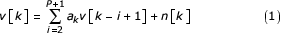where v[k] is the ECG time series, n[k] is zero mean white noise, a i 's are the AR coefficients, and P is the AR order.

A critical issue in AR modelling is the AR order used to model a signal. It is necessary to select an appropriate AR order so that the signal is modelled with sufficient accuracy so as to be useful for classification. Various model orders were used to estimate the accuracy of the reconstructed signals. The criteria used for evaluating the model order selection in this project were the correlation coefficient ρ and the signal-to-noise (SNR) ratio. The correlation coefficient ρ is computed using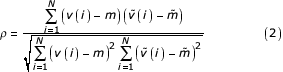where v(i) and(i) are the original and the simulated signals at the ith instant, and m and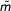are the mean of the original and simulated signals respectively and N is the length of the modeled signal. The signal-to-noise ratio is given by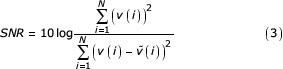Burg's algorithm was used to estimate the AR coefficients with a pre-selected model order P[23, 24].

### GLM-based classification

In the current study, AR coefficients were used to classify cardiac arrhythmias. A stage-by-stage GLM based classification model has been used for classification of the various cardiac arrhythmias. A GLM is given by= A β + ε           (4)

where= [y 1,y 2,...,y N ]T is an N-dimensional vector of observed responses, β = [β 0, β 1,...,β P ]T is a P+1 dimensional vector of unknown parameters, A is N × (P + 1) matrix of known predictors (AR coefficients) and ε = [ε 1,ε 2,...,ε N ]T is an N dimensional error vector.

The least squares estimator is given by

β = (AT A)-1 AT(5)

Generalized linear model based classification was performed in stages to differentiate between the normal ECG signals and the various cardiac arrhythmias. The various stages of classification and groupings in every stage are shown in Fig 1. During the training phase, the estimator β was computed based on the known classes of ECG segments that form the training set. The AR coefficients and the previously estimated β were used to compute the correct response at a particular stage of classification during the testing phase. To perform the stage by-stage classification, Euclidean distance measure between the AR coefficients of different classes was used to determine the groupings of classes at each stage. The AR coefficients [a(2),a(3),a(4),...,a(P+1)] of a particular ECG segment were mapped to a response (1 or -1) in every stage of classification. In the current study, the observation matrix A = [I, A2, A3, A4,..., Ap+1] where I is an identity vector and the column vectors A2, A3, A4,..., Ap+1 consist of AR coefficients a(2), a(3), a(4),...,a(p+1) respectively of all the ECG segments selected for training. The elements of vectorwere assigned values 1 or -1 depending on the membership of an ECG segment to a corresponding class or group. The number of elements inwas the number of examples in the training set. The estimator β was computed at each stage of classification based on the selected training sets. During testing, the output response (Y1 in stage 1, Y2 in stage 2, etc) was computed using the AR coefficients and the previously estimated β at each stage. A threshold value of zero was used to classify the output response as belonging to a group at a particular stage. Sixty samples from each class were used for training and the remaining was used for testing in the classification phase. The training sets were picked randomly and the sensitivity and specificity were measured for the NSR and the arrhythmias multiple times. The sensitivity and specificity was computed for all the classes as given by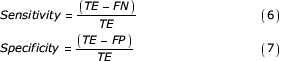where TE represents the total number of events, FN represents false negative, and FP represents false positive . The average sensitivity and specificity values were computed for NSR and the cardiac arrhythmias.

## Results

The AR modeling was applied to six different types of ECG signals from the MIT-BIH database. Classification was performed using a GLM-based classification algorithm.

### AR modeling results

Two main criteria, SNR and ρ were used to evaluate the performance of the AR model with different model orders. The correction coefficients for all ECG signals were 0.99. The SNR was calculated to be from 15.7 dB to 29.43 dB. Figure 2 shows the variation of SNR as a function of model order P. The SNR increased initially with model order P, but remains almost constant for model orders greater than or equal to four. In addition, computing the AR coefficients of higher orders would increase the number of computations. Hence, AR model of order four was used for further classification. The parameters computed using this model order were good enough to achieve a good SNR and correlation coefficient ρ and were found to be sensitive enough to differentiate the five types of ECG signals. The original NSR, APC, PVC, SVT, VT and VF segments as well as the modeled segments are shown in Figs 3,4,5,6,7 and 8.

The results were consistent with other studies on the selection of model order for AR modeling . AR modeling has been used for compression and it has been found that increase in accuracy by increasing the order of the predictor is negligible for predictors of order higher than 3 . The mean AR coefficients for all the ECG types used in the current study are shown in Table 1.

### Classification results

AR coefficients computed with order four were used for classification. Six types of ECG signals namely, NSR, APC, PVC, SVT, VT, and VF were considered for classification. Classification was performed using a generalized model linear model, which was applied in various stages. Figure 1 shows the stage-by-stage GLM-based classification algorithm for classifying various ECG signals. The six classes were separated into two groups with Normal, APC, PVC, and SVT signals forming one group and VT and VF forming another group at stage one. This grouping was evident by computing the Euclidean distance between the mean AR coefficients from various classes. The Euclidean distance between classes VF and VT was small. Similarly, the Euclidean distance among classes Normal, APC, PVC and SVT was small. The distance between VF/VT and Normal/APC/PVC/SVT was large and hence in the first stage, classes VT and VF were grouped together. The other group consisted of classes Normal, APC, PVC and SVT. In the second stage, VT and VT were differentiated. Stages three, four, five and six were used to differentiate between NSR, APC, PVC and SVT as shown in Fig 1.

The least squares estimator β was computed for various stages and the value Y was used to determine the classes in each stage. In the first stage (Y 1), the AR coefficients from an ECG signal was separated into two groups, one consisting of NSR, APC, PVC and SVT and the other consisting of VT and VF. In the second stage (Y 2), VT and VF were differentiated. In the third stage (Y 3), SVT was distinguished from NSR, APC and PVC. In the later stages (Y 4, Y 5 and Y 6), NSR, APV and PVC were distinguished from each other and classified.

The GLM was tested with 143 NSRs, 140 APCs, 155 PVCs, 143 VTs, 142 VFs, and 133 SVTs, which were obtained from the data sets by excluding the training data for each class. The sensitivity and specificity values were computed for all the ECG classes. The results of the GLM based classification are shown in Tables 2 and 3. The results for a sample training set are shown in Table 2 and the mean classification sensitivity and specificity for various classes are shown in Table 3. The accuracy of detecting NSR, APC, PVC, SVT, VT and VF were 93.2%, 96.4%, 94.8%, 100%, 97.7%, and 98.6% respectively.

## Discussion

Different values of AR modeling orders were tested for the ECG signals and the results showed that AR order of four is sufficient to model the ECG signal for the purpose of classification of selected arrhythmias. AR coefficients were used to classify the ECG beats into normal and five selected abnormal conditions. A stage-by-stage generalized linear model classification algorithm was used to distinguish between the different types of arrhythmias under consideration in the current study.

The classification results show that AR modeling can be used to discriminate between different arrhythmias. The classification results achieved using AR modeling is comparable to the recently published results on the classification of cardiac arrhythmias . Normal and abnormal PVC conditions have been classified using LPC coefficients classified using a fuzzy ARTMAP classifier with sensitivity of 97% and specificity of 99% . Accuracy of 93% and 96% has been reported for VT and VF respectively using a modified sequential probability ratio test algorithm . An overall accuracy of 93% to 99% was achieved with decimated ECG data and artificial neural networks . However, the data set consisted of a high number of NSR and PVC beats and the performance of beats including atrial premature beats was not very high. The total least squares-based Prony modeling technique produced an accuracy of 95.24%, 96% and 97.78% for SVT, VT and VF respectively .

AR modeling based classification algorithm has demonstrated good performance in classification. The algorithms are easy to implement and the AR coefficients can be easily computed. Preprocessing involves the detection of R peaks for which a number of techniques are available that can be implemented for real-time processing. A detailed comparison of computation times has not been performed; however, it is noted that computing the AR coefficients is simpler than most proposed measures for arrhythmia recognition. In addition, the computations were performed for 1.2 seconds of data only compared to 3 to 7 seconds for the complexity measures based technique  and 5 to 9 seconds in the Prony modeling technique .

Some of the proposed techniques use only a smaller number of arrhythmias (2–3) than the current study [2, 413, 15, 16]. The fuzzy ARTMAP technique has been used to classify normal and abnormal PVC conditions only . A time sequenced adaptive filter has been proposed for VT and VF alone . A real time discrimination algorithm with a Fourier-transform neural network has been has been proposed to distinguish between superventricular rhythms and ventricular rhythms in which PVC and VT were lumped together as belonging to a single class of ventricular rhythms . The complexity measure-based technique has been used to classify NSR, VT and VF . A QRS feature based-algorithm for decimated ECG data using artificial neural networks has been proposed that include various types of beats including APC and PVC, but they do not include the life threatening conditions like VT and VF . The Prony modeling technique has been used to classify SVT, VT and VF but their study does not include episodes from normal, APC or PVC . The current study classifies six different ECG classes and the performance is comparable to those studies that involve fewer classes.

In the current study, a fixed sample size has been used for AR modeling. A variable sample size based on the estimation of the R-R interval might yield better results independent of the heart rate of the subjects. The generalization capabilities of the AR model and the classification algorithms can be refined by applying the proposed approach to a larger data set. Further work is in progress to extend the proposed approach for classification of other types of cardiac arrhythmias as well as applying it to other signals of the cardiovascular system such as the hemodynamic signals, particularly for real-time applications. AR modeling is a linear modeling technique and might not necessarily be suitable for ECG signals under all conditions. Further work can be done to extend the current work to nonlinear parametric models that can better capture the non-linear and non-stationary nature of the ECG.

In addition to their utility in classification and diagnosis, AR coefficients can also be used for compression. AR modeling can lead to a low cost, high performance, simple to use portable telemedicine system for ECG offering a combination of diagnostic capability with compression.

## Conclusions

The proposed AR modeling and GLM for classification have been shown to be effective for the classification of cardiac arrhythmias in critically ill patients and aid in the diagnosis of heart disease. AR modeling and GLM models are suitable for real-time implementations and can be used for compression as well as diagnosis.

## References

1. Goldschlager N, Goldman MJ: Principles of Clinical Electrocardiography. Appleton and Lange 1989.

2. Barro S, Ruiz R, Cabello D, Mira J: Algorithmic sequential decision-making in the frequency domain for life threatening ventricular arrhythmias and imitative artefacts: a diagnostic system. J Biomed Eng 1989, 11: 320–328.

3. Coast DA, Stren RM, Cano GG, Briller SA: An approach to cardiac arrhythmia analysis using hidden Markov models. IEEE Trans Biomed Eng 1990, 37: 826–836. 10.1109/10.58593

4. Thakor NV, Zhu YS, Pan KY: Ventricular tachycardia and fibrillation detection by a sequential hypothesis testing algorithm. IEEE Trans Biomed Eng 1990, 37: 837–843. 10.1109/10.58594

5. Caswell SA, Kluge KS, Chiang CMJ: Pattern recognition of cardiac arrhythmias using two intracardiac channels. Proc Comp Cardiol 1993, 181–184.

6. Zhou SH, Rautaharju PM, Calhoun HP: Selection of a reduced set of parameters for classification of ventricular conduction defects by cluster analysis. Proc Comp Cardiol 1993, 879–882.

7. Afonoso VX, Tompkins WJ: Detecting ventricular fibrillation: Selecting the appropriate time-frequency analysis tool for the application. IEEE Eng Med Biol Mag 1995, 14: 152–159. 10.1109/51.376752

8. Ham FM, Han S: Classification of cardiac arrhythmias using fuzzy ARTMAP. IEEE Trans Biomed Eng 1996, 43: 425–430. 10.1109/10.486263

9. Finelli CJ: The time-sequenced adaptive filter for analysis of cardiac arrhythmias in intraventricular electrograms. IEEE Trans Biomed Eng 1996, 43: 811–819. 10.1109/10.508543

10. Chen SW, Clarkson PM, Fan Q: A robust sequential detection algorithm for cardiac arrhythmia classification. IEEE Trans Biomed Eng 1996, 43: 1120–1125. 10.1109/10.541254

11. Guvenir HA, Acar B, Demiroz G, Cekin A: A supervised learning algorithm for arrhythmia analysis. Comp Cardiol 1997, 24: 433–436.

12. Minami KC, Nakajima H, Toyoshima T: Real-time discrimination of ventricular tachyarrythmia with Fourier-transform neural network. IEEE Trans Biomed Eng 1999, 46: 179–185. 10.1109/10.740880

13. Xu SZ, Yi SZ, Thakor NV, Wang ZZ: Detecting ventricular tachycardia and fibrillation by complexity measure. IEEE Trans Biomed Eng 1999, 46: 548–555. 10.1109/10.759055

14. Melo SL, Caloba LP, Nadal J: Arrhythmia analysis using artificial neural network and decimated electrocardiographic data. Comp Cardiol 2000, 27: 73–76.

15. Small M, Yu DJ, Grubb N, Simonotto J, Fox KAA, Harrison RG: Automatic identification and recording of cardiac arrhythmia. Comp Cardiol 2000, 27: 355–358.

16. Chen SW: Two-stage discrimination of cardiac arrhythmias using a total least squares-based prony modeling algorithm. IEEE Trans Biomed Eng 2000, 47: 1317–1326. 10.1109/10.827310

17. Mukhopadhyay S, Sircar P: Parametric modelling of ECG signal. Med Biol Eng Comp 1996, 34: 171–173.

18. Pinna GD, Maestri R, Cesare AD: Application of time series spectral analysis theory: analysis of cardiovascular variability signals. Med Biol Eng Comp 1996, 34: 142–148.

19. Bennett FM, Chrisstini DJ, Ahmed H, Lutchen K: Time series modeling of heart rate dynamics. Proc Comp Cardiol 1993, 273–276.

20. Arnold M, Miltner WHR, Witte H: Adaptive AR modeling of nonstationary time series by means of Kalman filtering. IEEE Trans Biomed Eng 1998, 45: 553–562. 10.1109/10.668741

21. Mainardi LT, Bianchi AM, Baselli G, Cerutti S: Pole-tracking algorithms for the extraction of time-variant heart rate variability spectral parameters. IEEE Trans Biomed Eng 1995, 42: 250–258. 10.1109/10.364511

22. Lin KP, Chang WH: QRS feature extraction using linear prediction. IEEE Trans Biomed Eng 1989, 36: 1050–1055. 10.1109/10.40806

23. Marple SL: Digital spectral analysis with applications. Prentice Hall, Englewood Cliffs, New Jersey 1987.

24. Ljung L: System Identification: Theory for the user. Prentice Hall, Englewood Cliffs, New Jersey, 1999.

25. Anderson CW, Stolz EA, Shamssunder S: Multivariate autoregressive models for classification of spontaneous electroencephalographic signals during mental tasks. IEEE Trans Biomed Eng 1998, 45: 277–286. 10.1109/10.661153

26. Miller AS, Blott BH, Hames TK: Review of neural network applications in medical imaging and signal processing. Med Biol Eng Comp 1992, 30: 449–464.

27. Ramirez-Rodriguez CA, Hernandez-Silveira MA: Multi-thread implementation of a fuzzy neural network for automatic ECG arrhythmia detection. Comp Cardiol 2001, 28: 297–300.

28. Silipo R, Marchesi C: Artificial neural networks for automatic ECG analysis. IEEE Trans Sig proc 1998, 46: 1417–1425. 10.1109/78.668803

29. McCullagh P, Nelder JA: Generalized Linear Model. Chapman and Hall, London, 1989.

30. Tompkins W: Biomedical Digital Signal Processing. Prentice Hall, Englewood Cliffs, New Jersey 1993.

## Author information

Authors

### Corresponding author

Correspondence to Narayanan Srinivasan.

### Authors' contributions

DG carried out the analysis and implementation as well as testing of the software simulations. NS participated in the design and coordination of the study and testing of the software simulations. SMK conceived of the study and participated in its design and coordination.

All authors read and approved the final manuscript.

## Authors’ original submitted files for images

Below are the links to the authors’ original submitted files for images.

## Rights and permissions

Reprints and Permissions

Ge, D., Srinivasan, N. & Krishnan, S.M. Cardiac arrhythmia classification using autoregressive modeling. BioMed Eng OnLine 1, 5 (2002). https://doi.org/10.1186/1475-925X-1-5